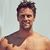# This Easy Math Quiz Is Actually Really Challenging

## Ambition is the path to success. Persistence is the vehicle you arrive in.Created by Michael Rogers
On Aug 26, 2021
1 / 15

4 ÷ 2 + 8 ÷ 4 = ?

2 / 15

½ of ½ of ½ of ½ of 64 = ?

3 / 15

4 + 4 × ¼ + 4 − 4 = ?

4 / 15

18 ÷ 3 + 18 ÷ 3 = ?

5 / 15

20 ÷ 4 x 10 ÷ 2 = ?

6 / 15

5 + 3 (5 + 3) = ?

7 / 15

1/3 of 1/3 of 99,999 equals:

8 / 15

2220/20 = ?

9 / 15

4 x 4 x 4 x 4 = ?

10 / 15

When I get multiplied by any number, the sum of the figures in the product is always me. What am I?

11 / 15

4 + 2 × 4 + 4 − 2 = ?

12 / 15

8 + 8 ÷ 8 + 8 x 8 – 8 = ?

13 / 15

10 x 1 + 10 ÷ 10 = ?

14 / 15

7 + 1 x 0 + 16 ÷ 2 = ?

15 / 15

9 ÷ 3 + 9 ÷ 3 = ?

15
Questions left
These are 10 of the World CRAZIEST Ice Cream Flavors
Created by Tal Garner
On Nov 18, 2021